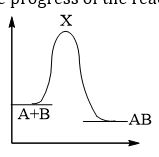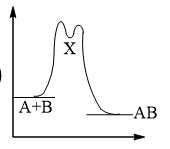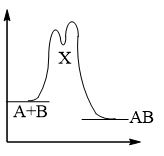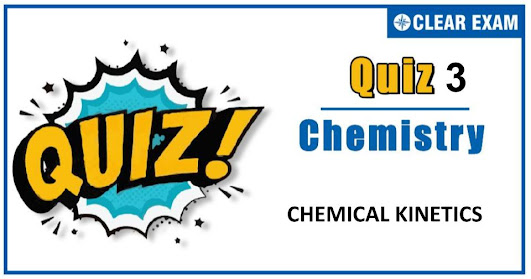## Q1. Effective collisions are those in which molecules must:  Have energy equal to or greater than the threshold energy  Have proper orientation  Acquire the energy of activation  All of the above Solution These are the characteristics of effective collisions Q2.Which of the following theory is not related to chemical kinetics?  Collision theory  VSPER theory  Activated complex theory  Absolute reaction rate theory Solution VSEPR theory is for bonding concept Q3.  What is the order of a reaction which has a rate expression rate =k[A]^(3/2) [B]^(-1)?   3/2  Zero  None of these  1/2 Solution C Q4. Among the following reaction, the fastest one is:  Burning of coal  Rusting of iron in moist air  Conversion of monoclinic sulphur to rhombic sulphur  Precipitation of silver chloride by mixing silver nitrate and sodium chloride solutions Solution Ionic reactions are instantaneous Q5.In a chemical reaction two reactants take part. The rate of reaction is directly proportion to the concentration of one of them and inversely proportional to the concentration of the other. The order of reaction is  0  1  2  4 Solution  The order of reaction is zero. Suppose the following reaction take place . A+B→product ∴rate=[A] [B]^(-1) ∴ order =1+(-1)=0 Q6. At 373 K, a gaseous reaction A→2B+C is found to be of first order. Starting with pure A, the total pressure at the end of 10 min was 176 mm and after a long time when A was completely dissociated, it was 270 mm. The pressure of A at the end of 10 min was  94 mm  47 mm 43 mm  90 mm SolutionQ7.An exothermic chemical reaction occurs in two steps as follows  a)b)c)All are correct Solution The reaction occurring in two steps has two activation energy peaks. The first step, being fast needs less activation energy. The second step being slow, needs more activation energy. Therefore, second peak will be higher than the first Q8.The activation energy of a reaction at a given temperature is found to be 2.303RT J mol^(-1) . The ratio of rate constant to the Arrhenius factor is  0.01  0.1  0.02  0.001 Solution Arrhenius equation is, Rate constant, k=Ae^(-E_a |RT) k=Ae^(-2.303 RT/RT) k/A=e^(-2.303) On solving, we get k/A=〖10〗^(-1) Q9.The unit of rate constant of second order reaction is  Mol/Ls  L^2/ mol^2  Per second  L / Mol s Solution The units of the rate constant for nth order reaction is (mol/L)^(1-n) s^(-1) ∴ for second order reaction, Unit of rate constant(mol/L)^(1-n) s^(-1) mol^(-1) Ls^(-1)=L /mol-s Q10. Consider a reaction; aG+bH→Products When concentration of both the reactants G and H is doubled, the rate increases by eight times. However, when concentration of G is doubled keeping the concentration of H fixed, the rate is doubled. The overall of the reaction,  0  3  1 2 Solution aG+bH→Products Suppose order of reaction =n When concentration of both G and H doubled then rate increases by eight times. rate=k(reactants)^n (8)=k(2)^n (2)^3=k(2)^n n=3 When concentration of G is doubled keeping the concentration of H fixed, the rate is doubled. Rate=[G]^1 then, Rate=[G]^1 [H]^2 var answers = ["D","B","D","D","A","B","C","B","D","B"]; var tot = answers.length; function getCheckedValue( radioName ){ var radios = document.getElementsByName( radioName ); //console.log(radios) for (var y=0; y < radios.length; y++){ if (radios[y].checked) return radios[y].value; } } function getScore(){ var score = 0; for (var i=0; i<tot; i++){ var answerVal = getCheckedValue('question'+i) //console.log(answerVal, answers[i]) if (typeof answerVal != undefined && answerVal == answers[i]) score += 1; } return score; } function returnScore(){ alert('Your score is' +getScore()+"/"+tot); } var q, m, s; function quizCount(){ if(!q){ console.log('mobile2'); m = 1, s = 0; } if(q){ clearTimeout(q); } m = 10, s = 0; \$('#timer').val(m + ":" + s); s = s-1; console.log(s) q = setTimeout("counter()", 1000); if(s<1){ m = m-1; console.log(m) s = 59; } } function counter(){ console.log(m); \$('#timer').val(m + ":" + s); s = s-1; console.log(s) q = setTimeout("counter()", 1000); if(s<1){ m = m-1; console.log(m) s = 59; } if(m<0){ quizStop(); } } function quizStop(){ clearTimeout(q); q= null; m = null; s = null; document.getElementById('timer').value= 'Quiz Finished'; alert("Your score is "+ getScore() +"/"+ tot); \$('.answer').slideDown(); \$('.opt-right').addClass('active'); \$('.start-btn, #reset').hide(); \$('#reset-start').show(); } function quizReset(){ clearTimeout(q); document.getElementById('timer').reset(); } \$(document).ready(function(){ // var a = \$('.question .q-options li .rad').length; \$('.submit').click(function(){ returnScore(); \$('.opt-right').addClass('active'); \$('.answer').slideDown(); }); \$('#reset').click(function(){ quizReset(); }); \$('#reset-start').click(function(){ \$('.opt-right').removeClass('active'); \$('.answer').slideUp(); \$('.start-btn, #reset').show(); \$('#reset-start').hide(); \$('.rad').prop('checked', false); }); }); { "@context": "https://schema.org/", "@type": "Quiz", "typicalAgeRange": "7-12", //ADD THE REQUIRED AGE RANGE "educationalLevel": "intermediate", //ADD THE DIFFICULTY "assesses": "chemical kinetics", //ADD THE TOPIC NAME "educationalAlignment": [ {"@type": "AlignmentObject", "alignmentType": "educationalSubject", "targetName": "chemistry" } //ADD THE TOPIC NAME IN &#8220;targetName&#8221; BLOCK,ADD &#8216;,&#8217;(COMMA) AND REPEAT THE HIGHLIGHTED //BLOCK FOR ADDING MORE TOPICS ], "name": "Quiz about chemical kinetics",//ADD THE //QUESTION TOPIC "about": { "@type": "Thing", "name": "chemical kinetics"//ADD THE QUESTION TOPIC }, "hasPart": [ { "@type": "Question", "eduQuestionType": "Multiple choice", //Question type "learningResourceType": "Practice problem", "name": "chemical kinetics-1",//ADD QUESTION NAME "text": " Effective collisions are those in which molecules must:",//ADD THE QUESTION TEXT "comment": { "@type": "Comment", "Text": "This is a hint of question"//ADD HINT FOR QUESTIONS }, "encodingFormat": "text/markdown", "suggestedAnswer": [ //HERE PUT THE WRONG ANSWERS/OPTIONS { "@type": "Answer", "position": 0,//OPTION POSITION 0,1,2,3 "encodingFormat": "text/markdown", "text": "Have energy equal to or greater than the threshold energy",//ADD THE ANSWER "comment": { "@type": "Comment", "text": "This is a hint" //COMMENT FOR ANSWER } }, { "@type": "Answer", "position": 1, "encodingFormat": "text/markdown", "text": "Have proper orientation", //ADD THE ANSWER "comment": { "@type": "Comment", "text": "This is a hint"//COMMENT FOR ANSWER } }, { "@type": "Answer", "position": 2, "encodingFormat": "text/markdown", "text": " Acquire the energy of activation",//ADD THE ANSWER "comment": { "@type": "Comment", "text": "This is a hint"//ADD COMMENT FOR ANSWER } } ], "acceptedAnswer": { //HERE PUT THE CORRECT ANSWER "@type": "Answer", "position": 3, "encodingFormat": "text/markdown", "text": "All of the above",//ADD THE ANSWER BETWEEN &#8220; &#8220; "comment": { "@type": "Comment", "text": "This is a hint"//ADD COMMENT FOR ANSWER } } }, { "@type": "Question", "eduQuestionType": "Multiple choice", //Question type "learningResourceType": "Practice problem", "name": "chemical kinetics-2",//ADD QUESTION NAME "text": "Which of the following theory is not related to chemical kinetics?",//ADD THE QUESTION TEXT "comment": { "@type": "Comment", "Text": "This is a hint of question"//ADD HINT FOR QUESTIONS }, "encodingFormat": "text/markdown", "suggestedAnswer": [ //HERE PUT THE WRONG ANSWERS/OPTIONS { "@type": "Answer", "position": 0,//OPTION POSITION 0,1,2,3 "encodingFormat": "text/markdown", "text": " Collision theory",//ADD THE ANSWER "comment": { "@type": "Comment", "text": "This is a hint" //COMMENT FOR ANSWER } }, { "@type": "Answer", "position": 2, "encodingFormat": "text/markdown", "text": " Activated complex theory", //ADD THE ANSWER "comment": { "@type": "Comment", "text": "This is a hint"//COMMENT FOR ANSWER } }, { "@type": "Answer", "position": 3, "encodingFormat": "text/markdown", "text": " Absolute reaction rate theory",//ADD THE ANSWER "comment": { "@type": "Comment", "text": "This is a hint"//ADD COMMENT FOR ANSWER } } ], "acceptedAnswer": { //HERE PUT THE CORRECT ANSWER "@type": "Answer", "position": 1, "encodingFormat": "text/markdown", "text": " VSPER theory",//ADD THE ANSWER BETWEEN &#8220; &#8220; "comment": { "@type": "Comment", "text": "This is a hint"//ADD COMMENT FOR ANSWER } } }, { "@type": "Question", "eduQuestionType": "Multiple choice", //Question type "learningResourceType": "Practice problem", "name": "chemical kinetics-3",//ADD QUESTION NAME "text": " What is the order of a reaction which has a rate expression \$\$ rate =k[A]^(3/2) [B]^(-1)\$\$ ?",//ADD THE QUESTION TEXT "comment": { "@type": "Comment", "Text": "This is a hint of question"//ADD HINT FOR QUESTIONS }, "encodingFormat": "text/markdown", "suggestedAnswer": [ //HERE PUT THE WRONG ANSWERS/OPTIONS { "@type": "Answer", "position": 0,//OPTION POSITION 0,1,2,3 "encodingFormat": "text/markdown", "text": "\$\$ 3/2\$\$",//ADD THE ANSWER "comment": { "@type": "Comment", "text": "This is a hint" //COMMENT FOR ANSWER } }, { "@type": "Answer", "position": 1, "encodingFormat": "text/markdown", "text": " Zero", //ADD THE ANSWER "comment": { "@type": "Comment", "text": "This is a hint"//COMMENT FOR ANSWER } }, { "@type": "Answer", "position": 2, "encodingFormat": "text/markdown", "text": "None of these",//ADD THE ANSWER "comment": { "@type": "Comment", "text": "This is a hint"//ADD COMMENT FOR ANSWER } } ], "acceptedAnswer": { //HERE PUT THE CORRECT ANSWER "@type": "Answer", "position": 3, "encodingFormat": "text/markdown", "text": "\$\$ 1/2\$\$",//ADD THE ANSWER BETWEEN &#8220; &#8220; "comment": { "@type": "Comment", "text": "This is a hint"//ADD COMMENT FOR ANSWER } } }, { "@type": "Question", "eduQuestionType": "Multiple choice", //Question type "learningResourceType": "Practice problem", "name": "chemical kinetics-4",//ADD QUESTION NAME "text": "Among the following reaction, the fastest one is:",//ADD THE QUESTION TEXT "comment": { "@type": "Comment", "Text": "This is a hint of question"//ADD HINT FOR QUESTIONS }, "encodingFormat": "text/markdown", "suggestedAnswer": [ //HERE PUT THE WRONG ANSWERS/OPTIONS { "@type": "Answer", "position": 0,//OPTION POSITION 0,1,2,3 "encodingFormat": "text/markdown", "text": "Burning of coal",//ADD THE ANSWER "comment": { "@type": "Comment", "text": "This is a hint" //COMMENT FOR ANSWER } }, { "@type": "Answer", "position": 1, "encodingFormat": "text/markdown", "text": "Rusting of iron in moist air", //ADD THE ANSWER "comment": { "@type": "Comment", "text": "This is a hint"//COMMENT FOR ANSWER } }, { "@type": "Answer", "position": 2, "encodingFormat": "text/markdown", "text": "Conversion of monoclinic sulphur to rhombic sulphur",//ADD THE ANSWER "comment": { "@type": "Comment", "text": "This is a hint"//ADD COMMENT FOR ANSWER } } ], "acceptedAnswer": { //HERE PUT THE CORRECT ANSWER "@type": "Answer", "position": 3, "encodingFormat": "text/markdown", "text": "Precipitation of silver chloride by mixing silver nitrate and sodium chloride solutions",//ADD THE ANSWER BETWEEN &#8220; &#8220; "comment": { "@type": "Comment", "text": "This is a hint"//ADD COMMENT FOR ANSWER } } }, { "@type": "Question", "eduQuestionType": "Multiple choice", //Question type "learningResourceType": "Practice problem", "name": "chemical kinetics-5",//ADD QUESTION NAME "text": "In a chemical reaction two reactants take part. The rate of reaction is directly proportion to the concentration of one of them and inversely proportional to the concentration of the other. The order of reaction is",//ADD THE QUESTION TEXT "comment": { "@type": "Comment", "Text": "This is a hint of question"//ADD HINT FOR QUESTIONS }, "encodingFormat": "text/markdown", "suggestedAnswer": [ //HERE PUT THE WRONG ANSWERS/OPTIONS { "@type": "Answer", "position": 1,//OPTION POSITION 0,1,2,3 "encodingFormat": "text/markdown", "text": "\$\$1\$\$",//ADD THE ANSWER "comment": { "@type": "Comment", "text": "This is a hint" //COMMENT FOR ANSWER } }, { "@type": "Answer", "position": 2, "encodingFormat": "text/markdown", "text": "\$\$ 2\$\$", //ADD THE ANSWER "comment": { "@type": "Comment", "text": "This is a hint"//COMMENT FOR ANSWER } }, { "@type": "Answer", "position": 3, "encodingFormat": "text/markdown", "text": "\$\$ 4\$\$",//ADD THE ANSWER "comment": { "@type": "Comment", "text": "This is a hint"//ADD COMMENT FOR ANSWER } } ], "acceptedAnswer": { //HERE PUT THE CORRECT ANSWER "@type": "Answer", "position": 0, "encodingFormat": "text/markdown", "text": "\$\$ 0\$\$",//ADD THE ANSWER BETWEEN &#8220; &#8220; "comment": { "@type": "Comment", "text": "This is a hint"//ADD COMMENT FOR ANSWER } } }, { "@type": "Question", "eduQuestionType": "Multiple choice", //Question type "learningResourceType": "Practice problem", "name": "chemical kinetics-6",//ADD QUESTION NAME "text": "\$\$At 373 K, a gaseous reaction A&#8594;2B+C is found to be of first order. Starting with pure A, the total pressure at the end of 10 min was 176 mm and after a long time when A was completely dissociated, it was 270 mm. The pressure of A at the end of 10 min was\$\$",//ADD THE QUESTION TEXT "comment": { "@type": "Comment", "Text": "This is a hint of question"//ADD HINT FOR QUESTIONS }, "encodingFormat": "text/markdown", "suggestedAnswer": [ //HERE PUT THE WRONG ANSWERS/OPTIONS { "@type": "Answer", "position": 0,//OPTION POSITION 0,1,2,3 "encodingFormat": "text/markdown", "text": "\$\$ 94 mm\$\$",//ADD THE ANSWER "comment": { "@type": "Comment", "text": "This is a hint" //COMMENT FOR ANSWER } }, { "@type": "Answer", "position": 2, "encodingFormat": "text/markdown", "text": "\$\$43 mm\$\$", //ADD THE ANSWER "comment": { "@type": "Comment", "text": "This is a hint"//COMMENT FOR ANSWER } }, { "@type": "Answer", "position": 3, "encodingFormat": "text/markdown", "text": "\$\$ 90 mm\$\$",//ADD THE ANSWER "comment": { "@type": "Comment", "text": "This is a hint"//ADD COMMENT FOR ANSWER } } ], "acceptedAnswer": { //HERE PUT THE CORRECT ANSWER "@type": "Answer", "position": 1, "encodingFormat": "text/markdown", "text": "\$\$ 47 mm\$\$",//ADD THE ANSWER BETWEEN &#8220; &#8220; "comment": { "@type": "Comment", "text": "This is a hint"//ADD COMMENT FOR ANSWER } } }, { "@type": "Question", "eduQuestionType": "Multiple choice", //Question type "learningResourceType": "Practice problem", "name": "chemical kinetics-7",//ADD QUESTION NAME "text": "An exothermic chemical reaction occurs in two steps as follows",//ADD THE QUESTION TEXT "comment": { "@type": "Comment", "Text": "This is a hint of question"//ADD HINT FOR QUESTIONS }, "encodingFormat": "text/markdown", "suggestedAnswer": [ //HERE PUT THE WRONG ANSWERS/OPTIONS { "@type": "Answer", "position": 0,//OPTION POSITION 0,1,2,3 "encodingFormat": "text/markdown", "text":" given image below", "image": "(https://1.bp.blogspot.com/-R0icvjvbmfc/X4cXKpQriNI/AAAAAAAAC3M/tDKKUz6wOBkiAY0aWRso_izdm5h3MkWnwCLcBGAsYHQ/s0/C27A.JPG)",//ADD THE ANSWER "comment": { "@type": "Comment", "text": "This is a hint" //COMMENT FOR ANSWER } }, { "@type": "Answer", "position": 1, "encodingFormat": "text/markdown", "text":" given image below", "image": "(https://1.bp.blogspot.com/-gV_9b8MPZaM/X4cXrJ-R3QI/AAAAAAAAC3U/9sUzO_18jLQwDjutSthlHU6KwpX_SWxKwCLcBGAsYHQ/s0/C27B.JPG)", //ADD THE ANSWER "comment": { "@type": "Comment", "text": "This is a hint"//COMMENT FOR ANSWER } }, { "@type": "Answer", "position": 3, "encodingFormat": "text/markdown", "text": "All are correct",//ADD THE ANSWER "comment": { "@type": "Comment", "text": "This is a hint"//ADD COMMENT FOR ANSWER } } ], "acceptedAnswer": { //HERE PUT THE CORRECT ANSWER "@type": "Answer", "position": 2, "encodingFormat": "text/markdown", "text":" given image below", "image": "(https://1.bp.blogspot.com/-IQXZf5WV7H4/X4cXvwQfkYI/AAAAAAAAC3Y/HWmGvB2UX_0cVXLQ58AcPInrp-wsBiYbACLcBGAsYHQ/s0/C27C.JPG)",//ADD THE ANSWER BETWEEN &#8220; &#8220; "comment": { "@type": "Comment", "text": "This is a hint"//ADD COMMENT FOR ANSWER } } }, { "@type": "Question", "eduQuestionType": "Multiple choice", //Question type "learningResourceType": "Practice problem", "name": "chemical kinetics-8",//ADD QUESTION NAME "text": "The activation energy of a reaction at a given temperature is found to be \$\$ 2.303RT J mol^(-1)\$\$ . The ratio of rate constant to the Arrhenius factor is",//ADD THE QUESTION TEXT "comment": { "@type": "Comment", "Text": "This is a hint of question"//ADD HINT FOR QUESTIONS }, "encodingFormat": "text/markdown", "suggestedAnswer": [ //HERE PUT THE WRONG ANSWERS/OPTIONS { "@type": "Answer", "position": 0,//OPTION POSITION 0,1,2,3 "encodingFormat": "text/markdown", "text": "\$\$ 0.01\$\$",//ADD THE ANSWER "comment": { "@type": "Comment", "text": "This is a hint" //COMMENT FOR ANSWER } }, { "@type": "Answer", "position": 2, "encodingFormat": "text/markdown", "text": "\$\$ 0.02", //ADD THE ANSWER "comment": { "@type": "Comment", "text": "This is a hint"//COMMENT FOR ANSWER } }, { "@type": "Answer", "position": 3, "encodingFormat": "text/markdown", "text": "\$\$ 0.001\$\$",//ADD THE ANSWER "comment": { "@type": "Comment", "text": "This is a hint"//ADD COMMENT FOR ANSWER } } ], "acceptedAnswer": { //HERE PUT THE CORRECT ANSWER "@type": "Answer", "position": 1, "encodingFormat": "text/markdown", "text": "\$\$ 0.1\$\$",//ADD THE ANSWER BETWEEN &#8220; &#8220; "comment": { "@type": "Comment", "text": "This is a hint"//ADD COMMENT FOR ANSWER } } }, { "@type": "Question", "eduQuestionType": "Multiple choice", //Question type "learningResourceType": "Practice problem", "name": "chemical kinetics-9",//ADD QUESTION NAME "text": "The unit of rate constant of second order reaction is ",//ADD THE QUESTION TEXT "comment": { "@type": "Comment", "Text": "This is a hint of question"//ADD HINT FOR QUESTIONS }, "encodingFormat": "text/markdown", "suggestedAnswer": [ //HERE PUT THE WRONG ANSWERS/OPTIONS { "@type": "Answer", "position": 0,//OPTION POSITION 0,1,2,3 "encodingFormat": "text/markdown", "text": "\$\$Mol/Ls\$\$",//ADD THE ANSWER "comment": { "@type": "Comment", "text": "This is a hint" //COMMENT FOR ANSWER } }, { "@type": "Answer", "position": 1, "encodingFormat": "text/markdown", "text": "\$\$L^2/ mol^2\$\$", //ADD THE ANSWER "comment": { "@type": "Comment", "text": "This is a hint"//COMMENT FOR ANSWER } }, { "@type": "Answer", "position": 2, "encodingFormat": "text/markdown", "text": "Per second",//ADD THE ANSWER "comment": { "@type": "Comment", "text": "This is a hint"//ADD COMMENT FOR ANSWER } } ], "acceptedAnswer": { //HERE PUT THE CORRECT ANSWER "@type": "Answer", "position": 3, "encodingFormat": "text/markdown", "text": "\$\$ L / Mol s\$\$",//ADD THE ANSWER BETWEEN &#8220; &#8220; "comment": { "@type": "Comment", "text": "This is a hint"//ADD COMMENT FOR ANSWER } } }, { "@type": "Question", "eduQuestionType": "Multiple choice", //Question type "learningResourceType": "Practice problem", "name": "chemical kinetics-10",//ADD QUESTION NAME "text": "\$\$Consider a reaction; aG+bH&#8594;Products When concentration of both the reactants G and H is doubled, the rate increases by eight times. However, when concentration of G is doubled keeping the concentration of H fixed, the rate is doubled. The overall of the reaction,\$\$",//ADD THE QUESTION TEXT "comment": { "@type": "Comment", "Text": "This is a hint of question"//ADD HINT FOR QUESTIONS }, "encodingFormat": "text/markdown", "suggestedAnswer": [ //HERE PUT THE WRONG ANSWERS/OPTIONS { "@type": "Answer", "position": 0,//OPTION POSITION 0,1,2,3 "encodingFormat": "text/markdown", "text": "\$\$ 0\$\$",//ADD THE ANSWER "comment": { "@type": "Comment", "text": "This is a hint" //COMMENT FOR ANSWER } }, { "@type": "Answer", "position": 2, "encodingFormat": "text/markdown", "text": "\$\$1\$\$", //ADD THE ANSWER "comment": { "@type": "Comment", "text": "This is a hint"//COMMENT FOR ANSWER } }, { "@type": "Answer", "position": 3, "encodingFormat": "text/markdown", "text": "\$\$2\$\$",//ADD THE ANSWER "comment": { "@type": "Comment", "text": "This is a hint"//ADD COMMENT FOR ANSWER } } ], "acceptedAnswer": { //HERE PUT THE CORRECT ANSWER "@type": "Answer", "position": 1, "encodingFormat": "text/markdown", "text": "\$\$3\$\$",//ADD THE ANSWER BETWEEN &#8220; &#8220; "comment": { "@type": "Comment", "text": "This is a hint"//ADD COMMENT FOR ANSWER } } } //ADD &#8216;,&#8217;(COMMA) AND REPEAT THE ABOVE HIGHLIGHTED PART,TO MARKUP MORE QUESTIONS ] }#### Written by: AUTHORNAME

AUTHORDESCRIPTION## Want to know more

Please fill in the details below:

## Latest NEET Articles\$type=three\$c=3\$author=hide\$comment=hide\$rm=hide\$date=hide\$snippet=hide

Name

ltr
item
BEST NEET COACHING CENTER | BEST IIT JEE COACHING INSTITUTE | BEST NEET, IIT JEE COACHING INSTITUTE: CHEMICAL KINETICS QUIZ-3
CHEMICAL KINETICS QUIZ-3
https://1.bp.blogspot.com/-iqgxK5NY0tE/X4cVVneBGRI/AAAAAAAAC28/yn4vOJY97hEEs8SKpAp4SaEgiUpUIwNpgCLcBGAsYHQ/w531-h376/Quiz%2BImage%2BTemplate%2B%252812%2529.jpg
https://1.bp.blogspot.com/-iqgxK5NY0tE/X4cVVneBGRI/AAAAAAAAC28/yn4vOJY97hEEs8SKpAp4SaEgiUpUIwNpgCLcBGAsYHQ/s72-w531-c-h376/Quiz%2BImage%2BTemplate%2B%252812%2529.jpg
BEST NEET COACHING CENTER | BEST IIT JEE COACHING INSTITUTE | BEST NEET, IIT JEE COACHING INSTITUTE
https://www.cleariitmedical.com/2020/10/chemical-kinetics-quiz-3.html
https://www.cleariitmedical.com/
https://www.cleariitmedical.com/
https://www.cleariitmedical.com/2020/10/chemical-kinetics-quiz-3.html
true
7783647550433378923
UTF-8